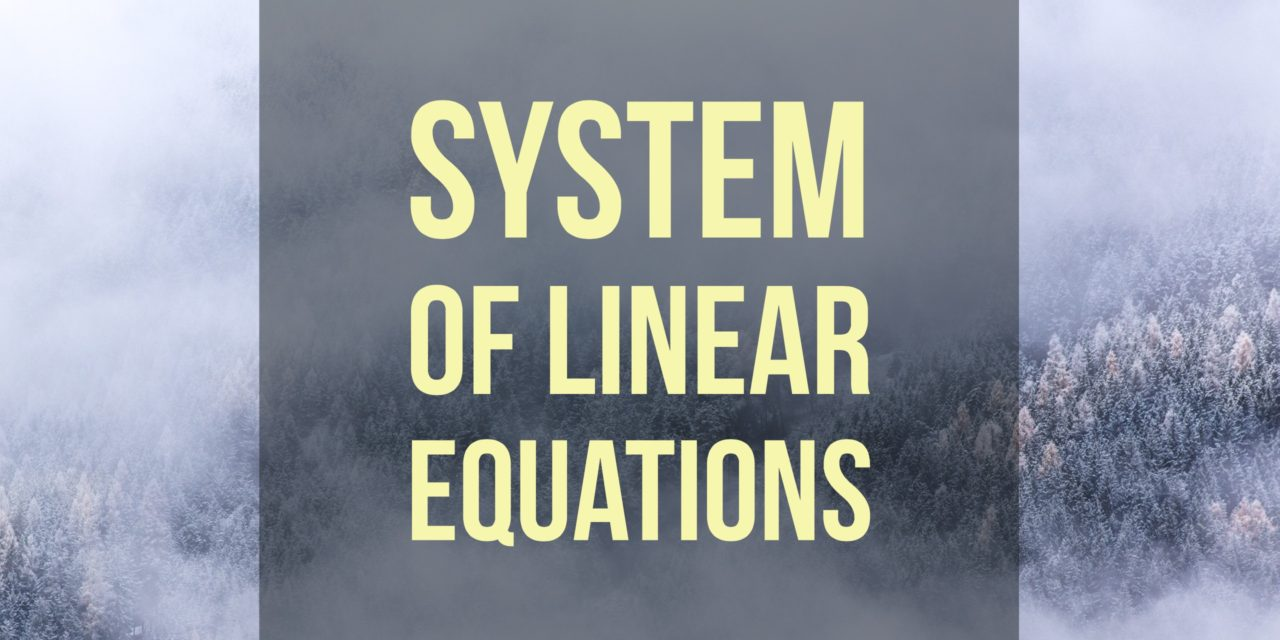# Linear Combination of Eigenvectors is Not an Eigenvector## Problem 258

Suppose that $\lambda$ and $\mu$ are two distinct eigenvalues of a square matrix $A$ and let $\mathbf{x}$ and $\mathbf{y}$ be eigenvectors corresponding to $\lambda$ and $\mu$, respectively.
If $a$ and $b$ are nonzero numbers, then prove that $a \mathbf{x}+b\mathbf{y}$ is not an eigenvector of $A$ (corresponding to any eigenvalue of $A$).Add to solve later

Contents

## Hint.

We use the following fact in the proof.
Fact: Two eigenvectors corresponding to distinct eigenvalues are linearly independent.

## Proof.

Seeking a contradiction, we assume that $a \mathbf{x}+b\mathbf{y}$ is an eigenvector corresponding to an eigenvalue $\zeta$.
Thus we have
\begin{align*}
A(a \mathbf{x}+b\mathbf{y})=\zeta (a \mathbf{x}+b\mathbf{y}). \tag{*}
\end{align*}

We calculate the left hand side of this equality as follows.
We have
\begin{align*}
A(a \mathbf{x}+b\mathbf{y})&=a A\mathbf{x}+bA\mathbf{y}\\
&=a\lambda \mathbf{x}+b\mu \mathbf{y}
\end{align*}
since $A\mathbf{x}=\lambda \mathbf{x}, A\mathbf{y}=\mu \mathbf{y}$ by defintion.

Therefore, from (*) we obtain
\begin{align*}
a(\lambda -\zeta) \mathbf{x}+b(\mu-\zeta)\mathbf{y}=\mathbf{0}.
\end{align*}

Recall that eigenvectors corresponding to distinct eigenvalues are linearly independent. Thus $\mathbf{x}$ and $\mathbf{y}$ are linearly independent.

Thus, the coefficients of the above linear combinations must be zero:
$a(\lambda -\zeta)=0 \text{ and } b(\mu-\zeta)=0.$

Since $a\neq0, b\neq 0$, this implies that we have
$\lambda=\zeta=\mu,$ and this is a contradiction because $\lambda$ and $\mu$ are supposed to be distinct.

Hence, $a \mathbf{x}+b\mathbf{y}$ cannot be an eigenvector of any eigenvalue of $A$.Add to solve later

### More from my site

#### You may also like...

This site uses Akismet to reduce spam. Learn how your comment data is processed.

###### More in Linear Algebra##### Use Cramer’s Rule to Solve a $2\times 2$ System of Linear Equations

Use Cramer's rule to solve the system of linear equations \begin{align*} 3x_1-2x_2&=5\\ 7x_1+4x_2&=-1. \end{align*}

Close